# Power BI：用DAX实现动态R-Visual

(本文将会讲解利用DAX度量值驱动R可视化的方法, 将会同时涉及DAX以及R语言相关知识)

### 前述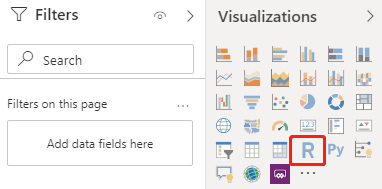### 效果

1. 实现绘制蜡烛图,网格线以及坐标轴 (R语言熟练者可跳过)
2. 在图中添加自定义辅助线或标记 (R语言熟练者可跳过)
3. 结合DAX, 实现用切片器控制R可视化, 实现深度交互(R+DAX 本文重点)

（注：关于蜡烛图，我开发了专门的可视化控件，详情点此

• 标红部分, 实现在切片器选择不同的颜色标准来切换蜡烛图的颜色, 中国标准为红涨绿跌, 国际标准与之相反.
• 标黄部分, 实现可以控制是否显示日均线以及日均线的切换(图中黄色曲线)
• 标蓝部分, 此部分可以实现让用户控制是否显示股市熔断的标记以及定点分析(此处还实现了判断所选股市是否有熔断机制,如果没有则不显示的效果), 其实现原理与前两个步骤相通, 本文不再赘述, 留读者自行发挥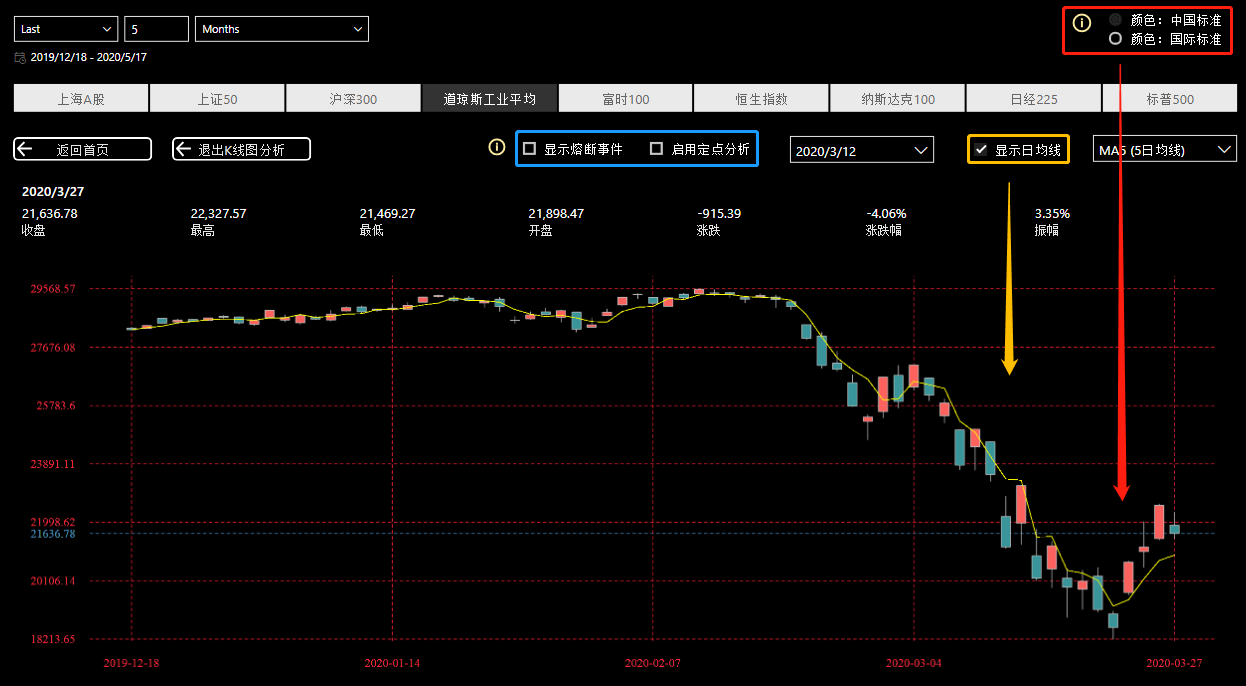#### 1.绘制蜡烛图, 网格线及坐标轴

(注: 本案例中, 五个字段名分别为: 日期: date 收盘价: CloseIndex 开盘价: OpenIndex 最高价: HighIndex 最低价: LowIndex 实际还有第六个字段: index_code, 这是指数代码, 在此是为了实现当用户选则不同的大盘指数时, 使图表可以使用对应的指数数据集,如果你的数据集里只有一种指数则不需要此字段. 以下代码,你只需将这六个字段的名称改成你自己的即可直接套用. 另外, 代码中有许多参数可供修改，比如颜色代码, 你可以改成你自己喜欢的颜色)

``````CODE <- dataset\$index_code
DATE<-dataset\$date

if(length(unique(CODE))>1)
{
dataset <- subset(dataset,dataset\$index_code == CODE[])
} else {
dataset = dataset
}

D<-dataset\$CloseIndex - dataset\$OpenIndex
N<-length(dataset\$date)
M <- N-11
w<-0.3
xIndex <- round(seq(from=1,to=N, na.rm=T,length=5))
xText <-substr(dataset\$date[xIndex],1,10)
yIndex <- seq(from=min(dataset\$LowIndex, na.rm=T),to=max(dataset\$HighIndex, na.rm=T),length=7)
yText <-round(yIndex,digits = 2)

left_margain <- if(max(nchar(yText))-3>0){0.5*max(nchar(yText)-3)+2} else {2}
bottom_margain <- 1.8
box_color = "grey"
par(mar=c(bottom_margain,left_margain,1,0.5)+0.5,family='serif',bg="transparent")

p <- plot(c(1:N),dataset\$CloseIndex,type = 'n',xaxt = 'n',yaxt='n', xlab = '', font.axis = 1.5,bty="n",ann=FALSE)+
abline(h = yText, lty = 2, col = "#FF2435")+
abline(v = xIndex, lty = 2, col = '#FF2435')+
for(i in 1:N)
{
lines(c(i,i),c(dataset\$LowIndex[i],dataset\$HighIndex[i]),col = box_color,lwd = 1)
x<-c(i-w,i-w,i+w,i+w)
y<-c(dataset\$OpenIndex[i],dataset\$CloseIndex[i],dataset\$CloseIndex[i],dataset\$OpenIndex[i])
if(D[i]<0)
{
polygon(x,y,col='#399599',border=box_color)
} else {
polygon(x,y,col='#FD625E',border=box_color)
}
} +
axis(side=1,at=xIndex,labels=xText,col.axis='#FF2435',tick=FALSE,las=1) +
axis(side=2,at=yIndex,labels=yText,col.axis='#FF2435',tick=FALSE,las=1)

p

``````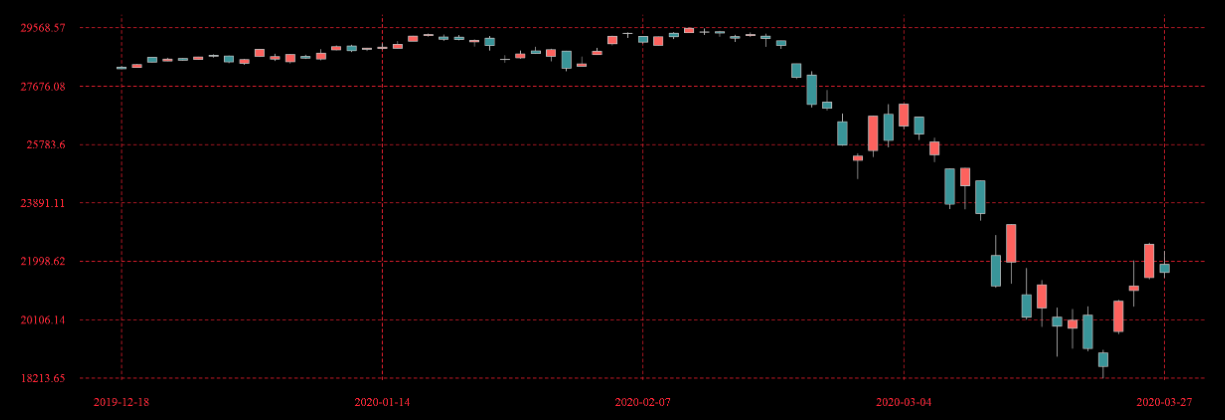#### 2. 添加自定义辅助线(或标记)

• 辅助线:
``````abline(h = dataset\$CloseIndex[N], lty = 2, col = 'SteelBlue')
``````
• 轴标签:
``````axis(side=2,at=dataset\$CloseIndex[N],labels=round(dataset\$CloseIndex[N],digits=2),
col.axis='SteelBlue',col.lab='green',tick=FALSE,las=1)
``````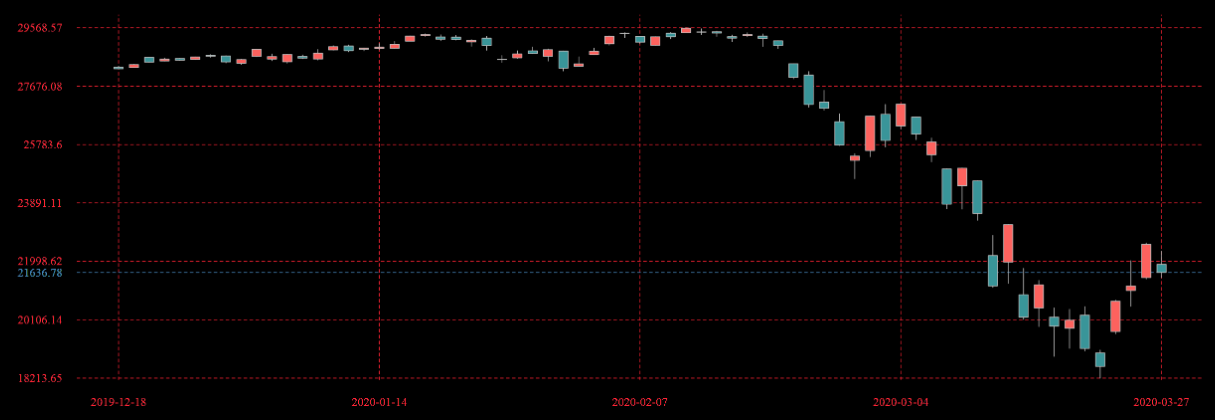#### 3. 利用DAX建立度量值控制R可视化

##### 基本原理案例: 日均线部分

``````MA_Value =
VAR SELECTED =
IF ( ISFILTERED ( PARA_MA[MA DAYS] ), MAX ( PARA_MA[DAYS] ), FALSE () )
VAR _MA =
SWITCH (
FALSE (),
BLANK(),
SELECTED, CALCULATE (
AVERAGEX (
FACT_STOCK,
CALCULATE ( AVERAGE(FACT_STOCK[close]), ALLEXCEPT ( FACT_STOCK, 'FACT_STOCK'[date],FACT_STOCK[index_code] ) )
),
DATESINPERIOD (
'FACT_STOCK'[date],
LASTDATE ( FACT_STOCK[date] ),
SELECTED*-1,
DAY
)
)
)
RETURN
IF(ISFILTERED(PARA_SHOW_MA[NAME]),_MA,BLANK())
``````

``````lines(c(1:N),dataset\$MA_Value,col="yellow",type='l',lwd=0.5)
``````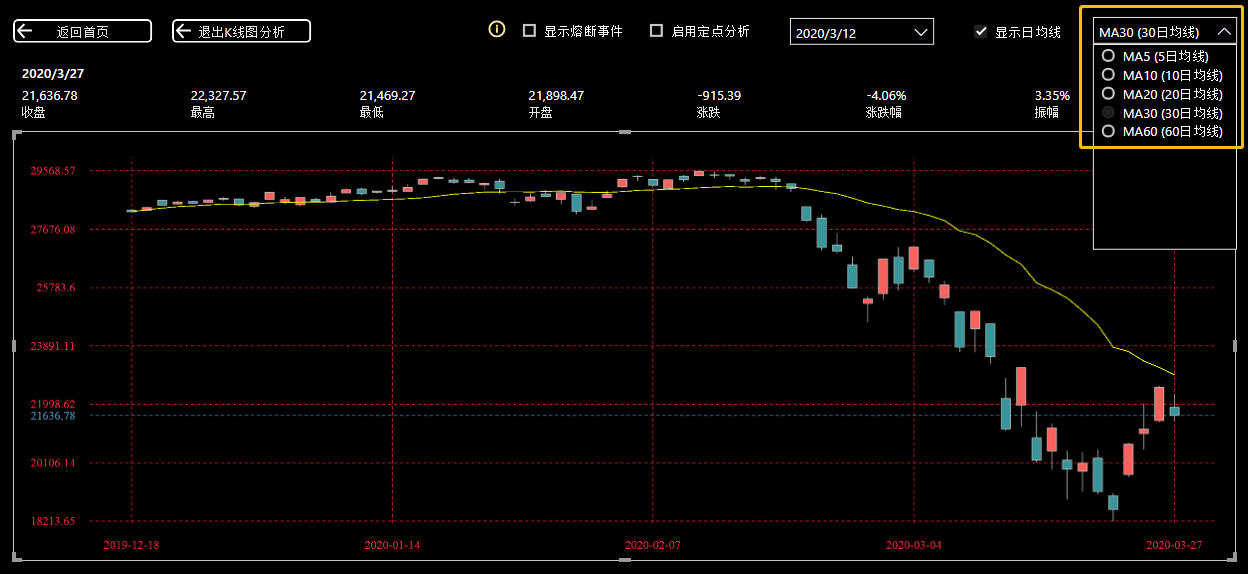(注: 此处利用公式中:IF(ISFILTERED(PARA_SHOW_MA[NAME]),_MA,BLANK())实现)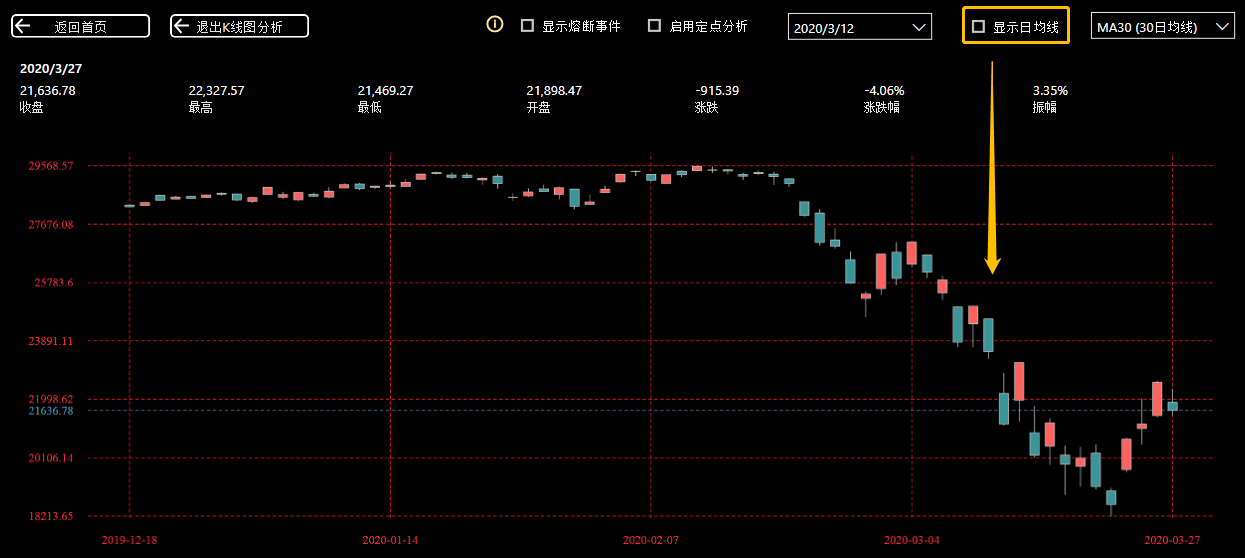##### 进阶案例: 切换颜色规则案例

``````ColorForRVisual_STOCK =
VAR _R = IF(
HASONEVALUE(PARA_COLORCONTROL[RULE]),VALUES(PARA_COLORCONTROL[VALUE]),BLANK()
)
RETURN
IF(_R,1,-1)
``````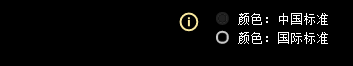``````ColorForRVisual_STOCK =
VAR _R =
IF(
HASONEVALUE(PARA_COLORCONTROL[RULE]),VALUES(PARA_COLORCONTROL[VALUE]),BLANK()
)
RETURN
IF(_R,1,-1)*'FACT_STOCK'[CloseIndex]
``````

`````` if(D[i]<0)
{
polygon(x,y,col='#399599',border=box_color)
} else {
polygon(x,y,col='#FD625E',border=box_color)
}
``````

`````` if(dataset\$ColorForRVisual_STOCK>0){
if(D[i]<0)
{
polygon(x,y,col='#399599',border=box_color)
} else {
polygon(x,y,col='#FD625E',border=box_color)
}
} else{
if(D[i]>=0)
{
polygon(x,y,col='#399599',border=box_color)
} else {
polygon(x,y,col='#FD625E',border=box_color)
}
}
``````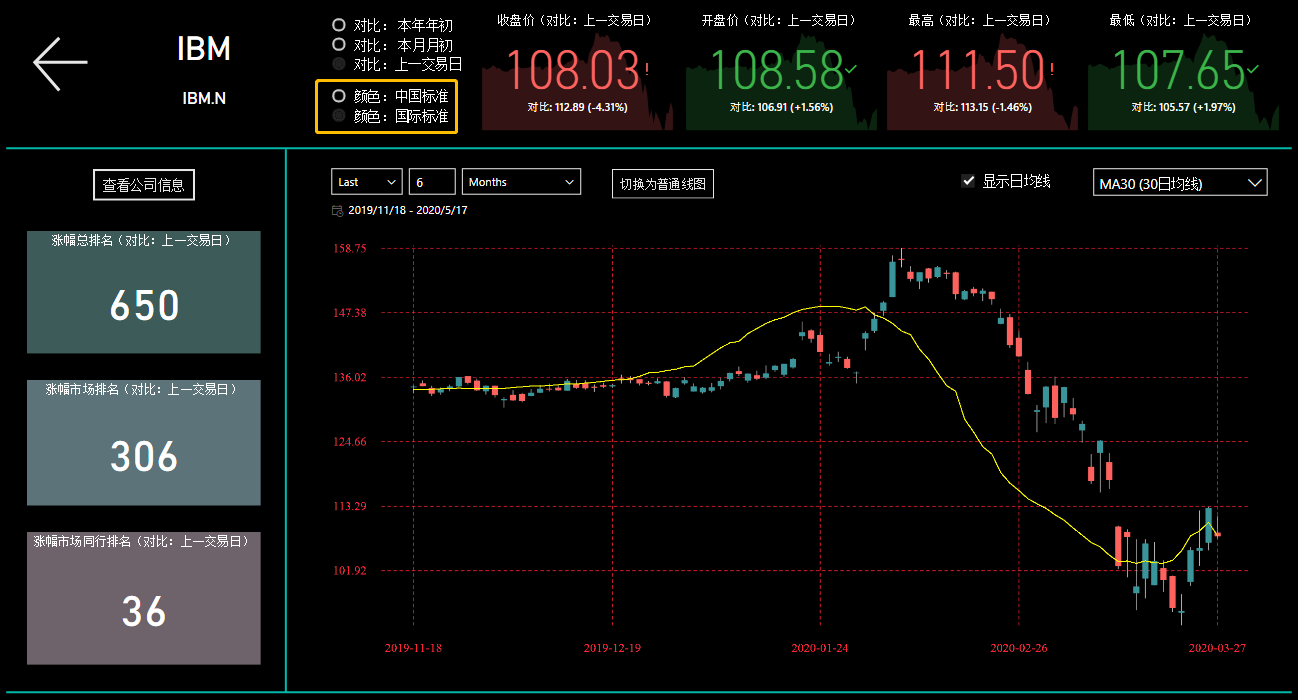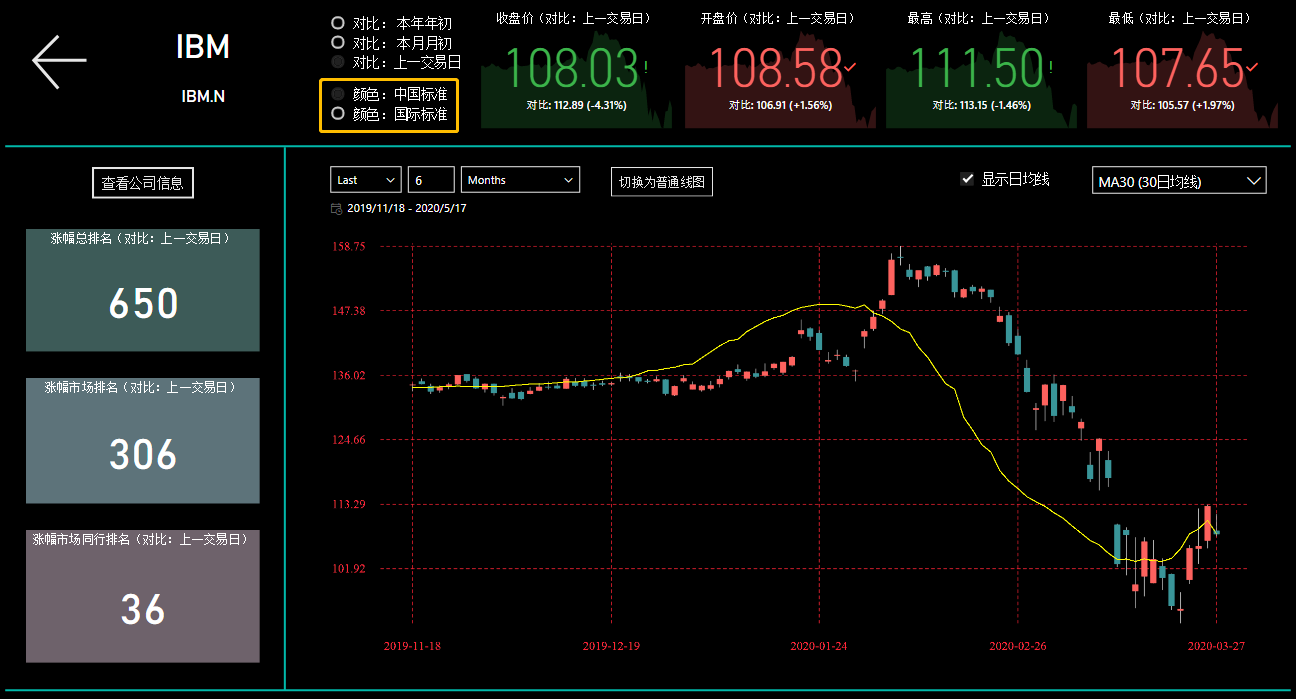### 总结

(本文涉及的可视化在此前已由本人分享至Power BI Community, 你可以在社区下载完整的R代码(点此到达) )